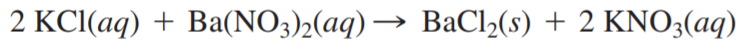×
Get Full Access to Chemistry: A Molecular Approach - 3 Edition - Chapter 4 - Problem 69e
Get Full Access to Chemistry: A Molecular Approach - 3 Edition - Chapter 4 - Problem 69e

×

# A 25.0 mL sample of a 1.20 M potassium chloride solutionISBN: 9780321809247 1

## Solution for problem 69E Chapter 4

Chemistry: A Molecular Approach | 3rd Edition

• Textbook Solutions
• 2901 Step-by-step solutions solved by professors and subject experts
• Get 24/7 help from StudySoup virtual teaching assistantsChemistry: A Molecular Approach | 3rd Edition

4 5 1 252 Reviews
31
0
Problem 69E

A 25.0 mL sample of a 1.20 M potassium chloride solution is mixed with 15.0 mL of a 0.900 M barium nitrate solution and this precipitation reaction occurs:The solid BaCl2 is collected, dried, and found to have a mass of 2.45 g. Determine the limiting reactant, the theoretical yield, and the percent yield.

Step-by-Step Solution:
Step 1 of 3

Solution: Here, we are going to identify the compound that will have highest concentration of potassium ion. Step1: a) 1 mole of KCl contains 1 mole of potassium(K ) ion + Therefore, 0.20 M KCL will contain 0.20 M K ion. + + Similarly, 0.15 M K CrO wil2conta4 (2 x 0.15 = 0.30) M K ion. And 0.080 M K PO will contain (3 x 0.080 = 0.24) M K ion. + 3 4 Thus, 0.15 M K CrO wi2 have4 ghest concentration of potassium ion. Step2: b) Number of moles of any compound in solution is given by: Number of moles of solute = Volume of solution in L X Molarity solute Therefore, number of moles of K CrO = (30.0 / 1000 L) X 0.15 M 2 4 = 0.030 L X 0.15 M = 0.0045 moles Similarly, number of moles of K PO = (25.0 / 1000 L) X 0.080 M 3 4 = 0.025 L X 0.080 M =0.002 moles. Now, 1 mole of K CrO will contain 2 moles of potassium ion. 2 4 Therefore, 0.0045 moles of K CrO will contain (2 x 0.0045 = 0.009) moles of potassium 2 4 ions. Similarly, 0.002 moles of K PO will co3ain43 x 0.002 = 0.006) moles of potassium ions. Thus, 30.0 ml of 0.15 M K CrO contai2 great4 number of moles of potassium ion. --------------------

Step 2 of 3

Step 3 of 3

##### ISBN: 9780321809247

This textbook survival guide was created for the textbook: Chemistry: A Molecular Approach, edition: 3. The answer to “?A 25.0 mL sample of a 1.20 M potassium chloride solution is mixed with 15.0 mL of a 0.900 M barium nitrate solution and this precipitation reaction occurs: The solid BaCl2 is collected, dried, and found to have a mass of 2.45 g. Determine the limiting reactant, the theoretical yield, and the percent yield.” is broken down into a number of easy to follow steps, and 54 words. Chemistry: A Molecular Approach was written by and is associated to the ISBN: 9780321809247. This full solution covers the following key subjects: ion, potassium, cro, concentration, Greater. This expansive textbook survival guide covers 82 chapters, and 9454 solutions. The full step-by-step solution to problem: 69E from chapter: 4 was answered by , our top Chemistry solution expert on 02/22/17, 04:35PM. Since the solution to 69E from 4 chapter was answered, more than 1175 students have viewed the full step-by-step answer.

Unlock Textbook Solution